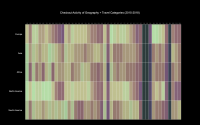2D Library Checkout Activity
MAT 259, 2018
Wilson Mui

Concept
In this project, I wanted to visualize the checkout activity for Dewey classes 914-918 over a four year period. The goal was to create a visualization that could easily show checkout activity over a period of time. Hopefully, it would make changes in checkout activity very clear to viewers. Dewey classes in 910 relate to geography and travel. The classes 914-918 refer to Europe, Asia, Africa, North America, and South America. The changes in checkouts is mainly shown through the use of color. For the colors, each row also is independent of the others. This means that the checkout volume may vary greatly between different categories, but the color will not represent this. To show the difference in checkout volume, the font size of the categories is changed. Bigger font will mean that there are more checkouts overall. Pressing numbers 0-4 would change the colors used. Some color schemes have a much greater difference in color, and this could make the changes in checkouts more apparent. Hovering the mouse over a colored square would display the number of checkouts for the category over that month.

Query
SELECT SUBSTR(deweyClass, 1, 3) AS dClass, SUM(IF(MONTH(cout) = 1, 1, 0)) AS Jan, SUM(IF(MONTH(cout) = 2, 1, 0)) AS Feb, SUM(IF(MONTH(cout) = 3, 1, 0)) AS Mar, SUM(IF(MONTH(cout) = 4, 1, 0)) AS Apr, SUM(IF(MONTH(cout) = 5, 1, 0)) AS May, SUM(IF(MONTH(cout) = 6, 1, 0)) AS Jun, SUM(IF(MONTH(cout) = 7, 1, 0)) AS Jul, SUM(IF(MONTH(cout) = 8, 1, 0)) AS Aug, SUM(IF(MONTH(cout) = 9, 1, 0)) AS Sep, SUM(IF(MONTH(cout) = 10, 1, 0)) AS Oct, SUM(IF(MONTH(cout) = 11, 1, 0)) AS Nov, SUM(IF(MONTH(cout) = 12, 1, 0)) AS 'Dec' FROM spl_2016.outraw WHERE (FLOOR(deweyClass) = 914 OR FLOOR(deweyClass) = 915 OR FLOOR(deweyClass) = 916 OR FLOOR(deweyClass) = 917 OR FLOOR(deweyClass) = 918) AND YEAR(cout) BETWEEN 2014 AND 2018 GROUP BY dClass;

Final result
Different color schemes may be better at revealing patterns and discrepencies.Code
All work is developed within Processing
Source Code + Data